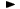ADVFN HomeHelpFinancialsDeeper AnalysisFINANCIAL RATIOS - Liquidity RatiosCash & Equv./Current Assets

# Cash & Equv./Current Assets

Cash & Equivalents to Current Assets percentage, is the cash & equivalents divided by the total current assets i.e. the total of all other current assets, stocks, debtors and cash & equivalents.

The formula is the following:

= [cash & equivalents / (current assets,other + stocks + debtors + cash & equivalents)] * 100